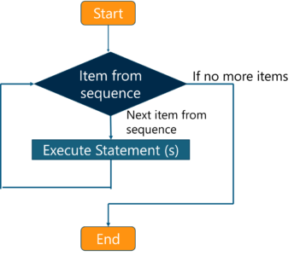# Important JavaScript Loops You Need to KnowA Data Science Enthusiast with in-hand skills in programming languages such as...

Loops in JavaScript is used for making decisions by validating a particular value against a given test condition and execute a particular code segment for a specific number of times until the given condition is satisfied. It helps in making your code compact. The JavaScript loops are explained in this article in the following sequence:

## JavaScript Loops

JavaScript loops provide a quick and easy method of doing something repeatedly. They are used to repeat an action number of times without having to repeat the same line of code. There are mainly two types of loops:

• Entry Controlled Loops – In entry controlled, the test condition is tested before entering the loop body. For Loop and While Loop are entry controlled loops.

• Exit Controlled Loops- In exit controlled, the test condition is evaluated at the end of the loop body. Therefore, the loop body will execute at least once, irrespective of whether the test condition is true or false. do-while loop is an exit controlled loop.

Now let’s move on and discuss the different methods for executing JavaScript loops.

## For Loop

This method provides a concise way of writing the loop structure. A for loop repeats until a specified condition evaluates to false.Syntax-

```
for (initialization condition; testing condition; increment/decrement)
{
statement
}

```

Example-

```
<script type = "text/javaScript">
// JavaScript program to illustrate for loop
var x;
// for loop begins when x=1
// and runs till x <=5
for (x = 1; x <= 5; x++)
{
document.write("Value of x:" + x + "<br />");
}
< /script>

```

Output-

```Value of x:1
Value of x:2
Value of x:3
Value of x:4```

## While Loop

A while loop is a control flow statement which allows the code to be executed repeatedly based on a particular Boolean condition. This loop is similar to that of a repeating if statement.Syntax-

```
while (boolean condition)
{
loop statements
}

```

Example-

```
<script type = "text/javaScript">
// JavaScript program to illustrate while loop
var x = 1;
// Exit when x becomes greater than 5
while (x <= 5)
{
document.write("Value of x:" + x + "<br />");
// increment the value of x for
// next iteration
x++;
}
< /script>

```

Output-

```Value of x:1
Value of x:2
Value of x:3
Value of x:4
Value of x:5```

### Do-while Loop

This loop is similar to while loop but the only difference is that it checks for condition after executing the statements. Thus, it is an example of Exit Controlled Loop.Syntax-

```
do
{
statements
}
while (condition);

```

Example-

```
<script type = "text/javaScript">
// JavaScript program to illustrate do-while loop
var x = 20;
do
{
// if the condition is false
document.write("Value of x:" + x + "<br />");
x++;
} while (x < 10);
< /script>

```

Output-

`Value of x: 20`

For-in Loop

This loop iterates a specified variable over all the enumerable properties of an object. For each distinct property, JavaScript will execute the specified statements.

Syntax-

```
for (variableName in Object)
{
statements
}

```

Example-

```
<script type = "text/javaScript">
// JavaScript program to illustrate for..in loop
// creating an Object
var characteristic = { first : "Name", second : "Age",
third : "Height", fourth : "Eye-color",
fifth : "Nationality" };
// iterate through every property of the
// object characteristics and print all of them
// using for..in loops
for (itr in characteristics)
{
document.write(characteristics[itr] + "<br >");
}
< /script>

```

Output-

```Name
Age
Height
Eye-color
Nationality```

## Break Statement

The break statement is used for jumping out of a loop. It will help you in breaking the loop and continue executing the code after the loop.

Syntax-

```
break labelname;

```

Example-

```
var text = ""
var i;
for (i = 0; i < 10; i++) {
if (i === 5) {
break;
}
text += "The number is " + i + "<br>";
}

```

Output-

```The number is 0
The number is 1
The number is 2
The number is 3
The number is 4```

## Continue Statement

The continue statement breaks one iteration in the loop if a specified condition occurs, and continues with the next iteration in the loop. The difference between continue and the break statement is that the continue statement “jumps over” one iteration in the loop instead of “jumping out”. For more check out this full stack development course today.

Syntax-

```
continue labelname;

```

Example-

```
var text = ""
var i;
for (i = 0; i < 5; i++) {
if (i === 2) {
continue;
}
text += "The number is " + i + "<br>";
}

```

Output-

```The number is 0
The number is 1
The number is 3
The number is 4```

These were the different methods of executing JavaScript Loops. With this we have come to the end of our article.

Now that you know about JavaScript Loops, check out the Web Development Certification Training by Edureka. Web Development Certification Training will help you Learn how to create impressive websites using HTML5, CSS3, Twitter Bootstrap 3, jQuery and Google APIs and deploy it to Amazon Simple Storage Service(S3).

Got a question for us? Please mention it in the comments section of “JavaScript Loops” and we will get back to you.

Upcoming Batches For Java Certification Training Course
Course NameDate
Java Certification Training Course

Class Starts on 29th April,2023

29th April

SAT&SUN (Weekend Batch)
View DetailsREGISTER FOR FREE WEBINARThank you for registering Join Edureka Meetup community for 100+ Free Webinars each month

### Subscribe to our Newsletter, and get personalized recommendations.#### Important JavaScript Loops You Need to Know

edureka.co